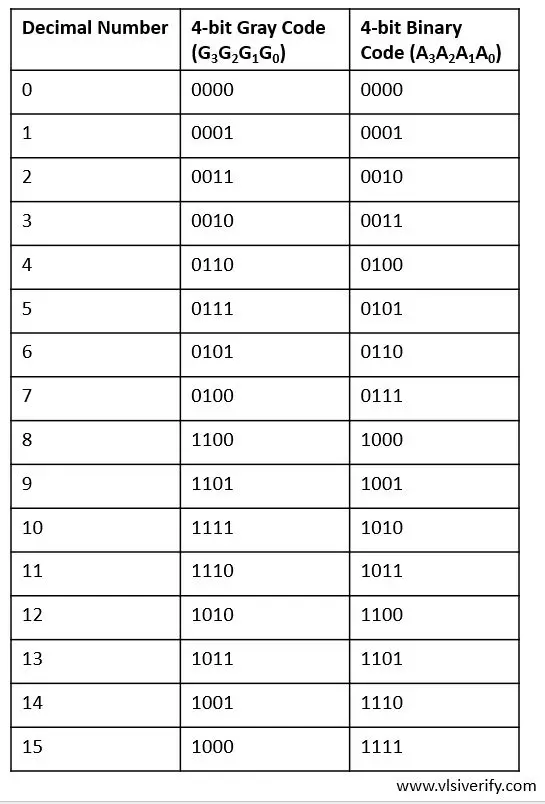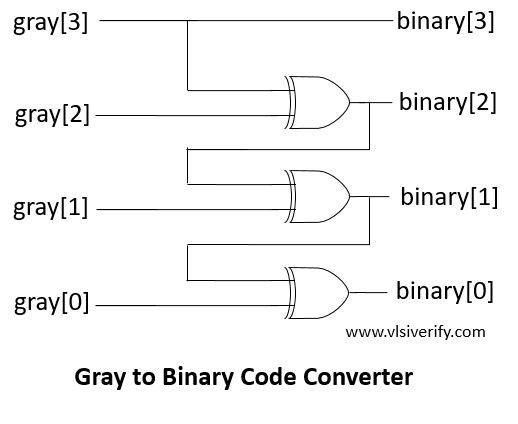Tutorials

Gray code has its own applications and we have seen how binary code is converted to the Gray code in the previous post Binary to Gray Code Converter.

Let’s Gray code to Binary code implementation in Verilog.

## Truth TableBy solving using K-map technique, a simple combinational circuit turned out as## Gray to Binary Code Converter Verilog code

``````module g2b_converter #(parameter WIDTH=4) (input [WIDTH-1:0] gray, output [WIDTH-1:0] binary);
/*
assign binary = gray ^ gray ^ gray ^ gray;
assign binary = gray ^ gray ^ gray;
assign binary = gray ^ gray;
assign binary = gray;
*/
// OR
genvar i;
generate
for(i=0;i<WIDTH;i++) begin
assign binary[i] = ^(gray >> i);
end
endgenerate
endmodule``````

### Testbench Code

``````module TB;
reg [3:0] binary, gray;
g2b_converter g2b(gray, binary);

initial begin
\$monitor("Gray = %b --> Binary = %b", gray, binary);
gray = 4'b1110; #1;
gray = 4'b0100; #1;
gray = 4'b0111; #1;
gray = 4'b1010; #1;
gray = 4'b1000;
end
endmodule``````

Output:

``````Gray = 1110 --> Binary = 1011
Gray = 0100 --> Binary = 0111
Gray = 0111 --> Binary = 0101
Gray = 1010 --> Binary = 1100
Gray = 1000 --> Binary = 1111``````

Verilog Codes